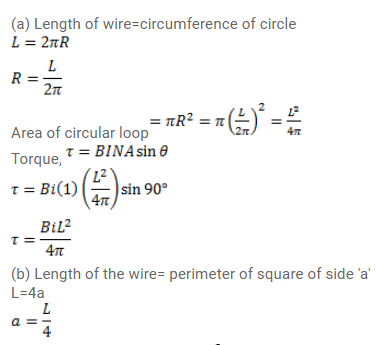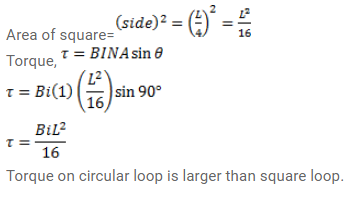# A circular loop carrying a current i has wire of total length

Question:

A circular loop carrying a current i has wire of total length $\mathrm{L}$. A uniform magnetic field B exists parallel to the plane of the loop.

(a) Find the torque on the loop.

(b) If the same length of the wire is used to form a square loop, what would be the torque? Which is larger?

Solution: